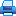Legal and postal addresses of the publisher: office 1410a, 17 Naberezhnaya Severnoy Dviny, Arkhangelsk, 163002, Russian Federation, Northern (Arctic) Federal University named after M.V. Lomonosov

Phone: (818-2) 21-61-21
E-mail: vestnik_est@narfu.ru
http://aer.narfu.ru/en/

# High-Frequency Asymptotics of Solutions of Some Linear Differential Equations with Periodic Coefficients. P. 129–133Section: Physics. Mathematics. Informatics

517.925

### Authors

Tomashevsky Igor Ludvigovich
Institute of Mathematics, Information and Space Technologies, Northern (Arctic) Federal University named after M.V. Lomonosov (Arkhangelsk, Russia)
е-mail: tomashevil@gmail.com

### Abstract

The paper studied some class of systems of n linear differential equations with T- periodic coefficients. Analytical method of asymptotically exact solution of these systems, when T →0 , was described, and a simple algorithm to calculate Floquet exponents of their fundamental solutions was obtained. The algorithm reduces the search of fundamental solutions of the original combined equations to a problem on eigenvalues and eigenelements of some self-adjoint H operator acting in a special type of Hilbert space. Perturbation theory, using T as a small parameter, transforms the spectral problem for H operator into a problem on eigenvalues of some square matrix of order n. Eigenvalues of this matrix represent approximate values of Floquet exponents of fundamental solutions of the original system. The obtained approximate values of Floquet exponents were compared to the results of “exact” numerical calculations. An upper estimate for the maximum error of approximate values was obtained. Explicit analytical expressions for approximate values of Floquet exponents, when n = 2 , were given.

### Keywords

linear differential equations with periodic coefficients, Floquet exponent, characteristic exponent